What is Matter Mass Mass and Weight Weight

• Slides: 52What is Matter?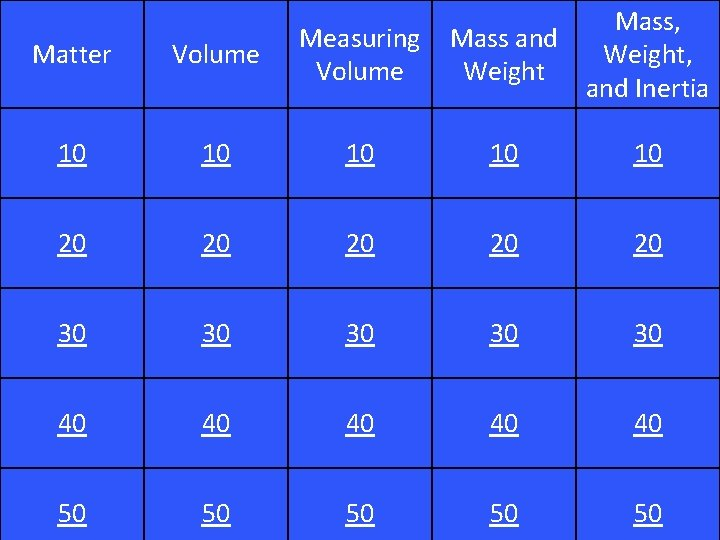Mass, Mass and Weight, Weight and Inertia Matter Volume Measuring Volume 10 10 10 20 20 20 30 30 30 40 40 40 50 50 50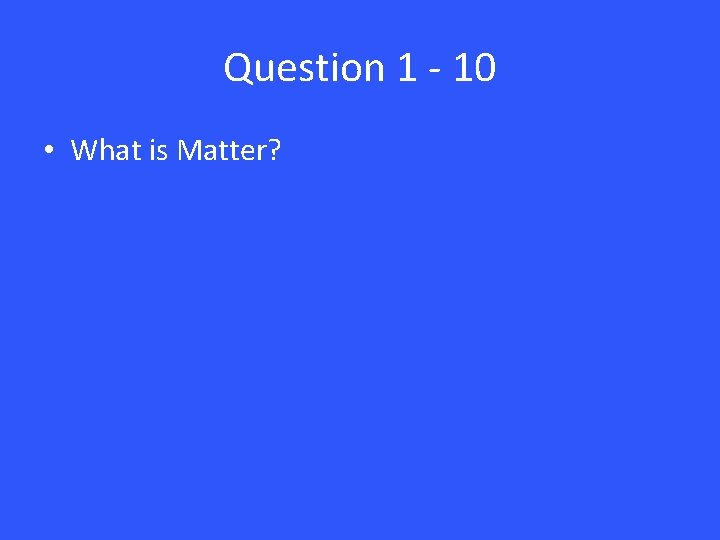Question 1 - 10 • What is Matter?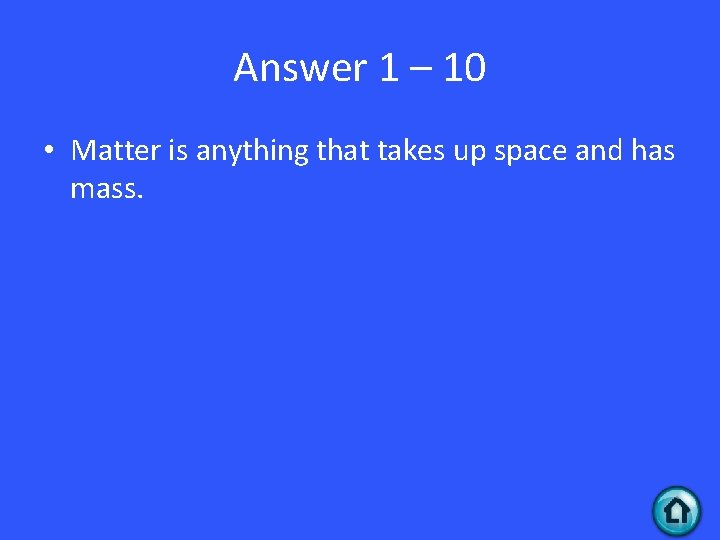Answer 1 – 10 • Matter is anything that takes up space and has mass.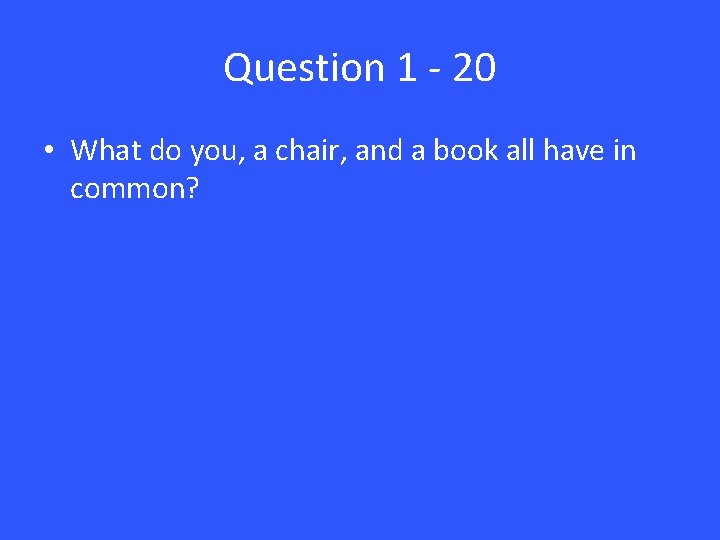Question 1 - 20 • What do you, a chair, and a book all have in common?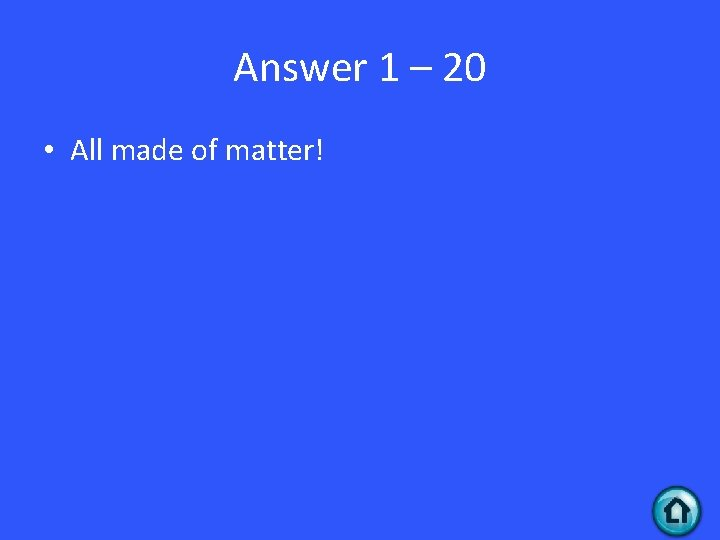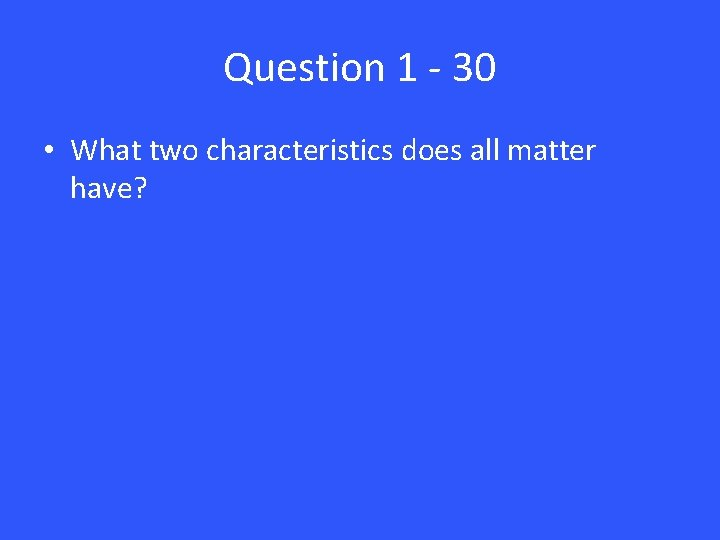Question 1 - 30 • What two characteristics does all matter have?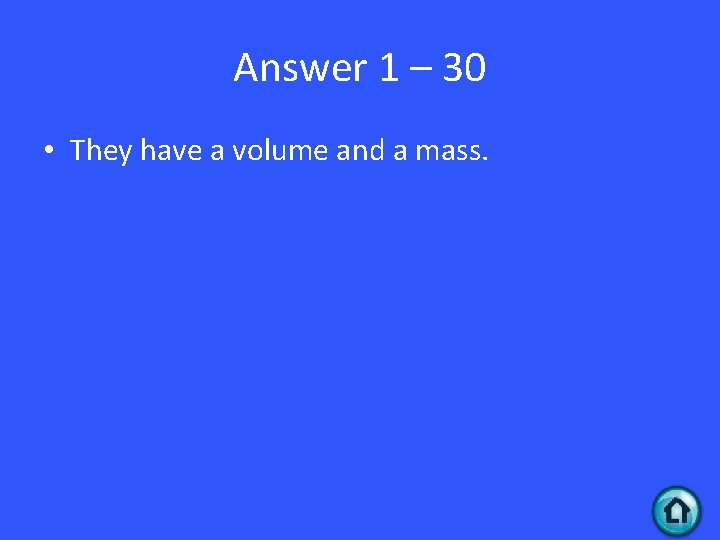Answer 1 – 30 • They have a volume and a mass.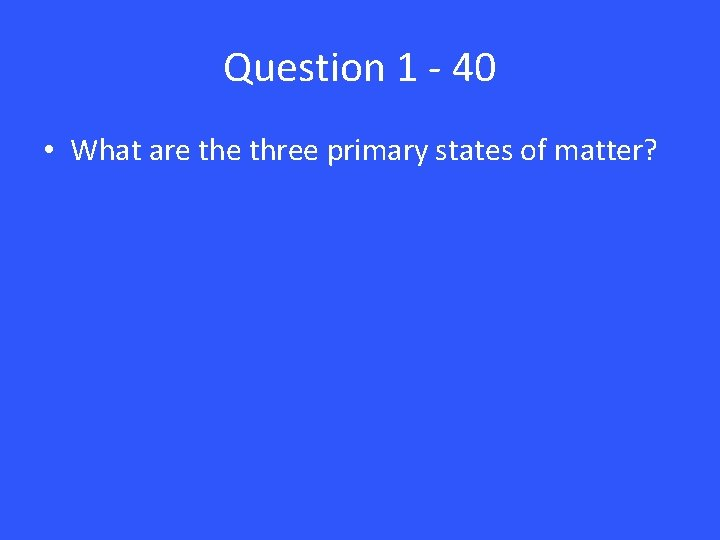Question 1 - 40 • What are three primary states of matter?Answer 1 – 40 • Solid, Liquid, and GasQuestion 1 - 50 • Explain how the particles of a solid behave.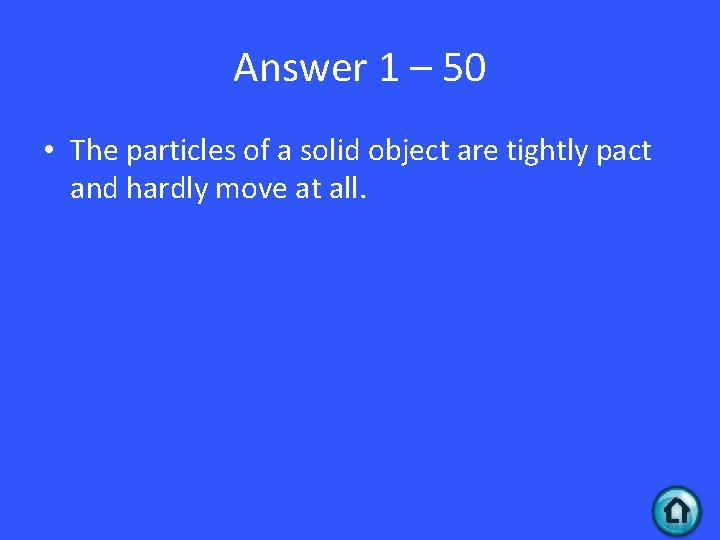Answer 1 – 50 • The particles of a solid object are tightly pact and hardly move at all.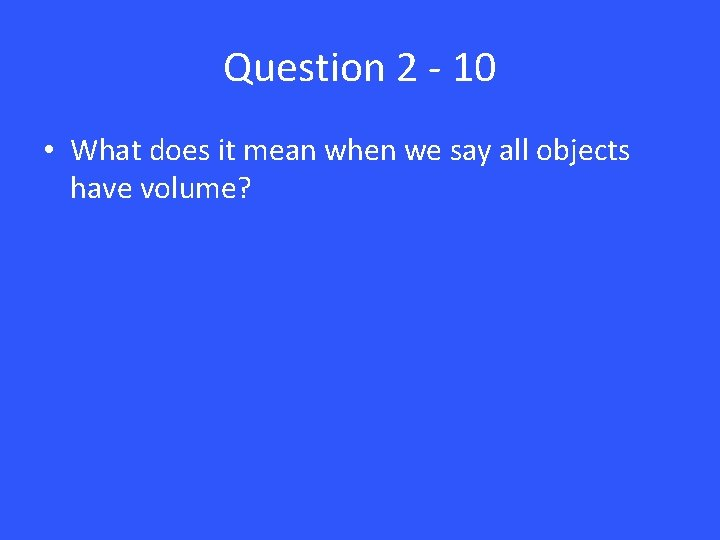Question 2 - 10 • What does it mean when we say all objects have volume?Answer 2 – 10 • All objects take up some amount of space.Question 2 - 20 • True or False: Two objects are able to be in the same exact space at the same time.Answer 2 – 20 • False!Question 2 - 30 • What SI unit is traditionally used to measure LIQUID volume?Answer 2 – 30 • Liters (L) and milliliters (m. L)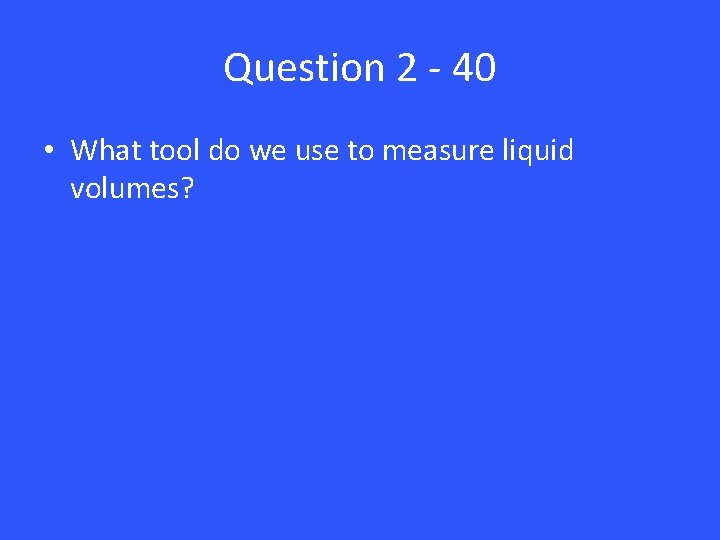Question 2 - 40 • What tool do we use to measure liquid volumes?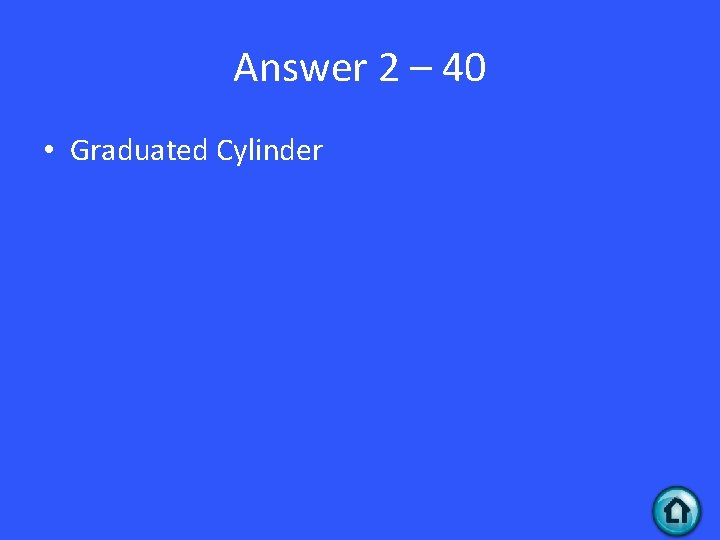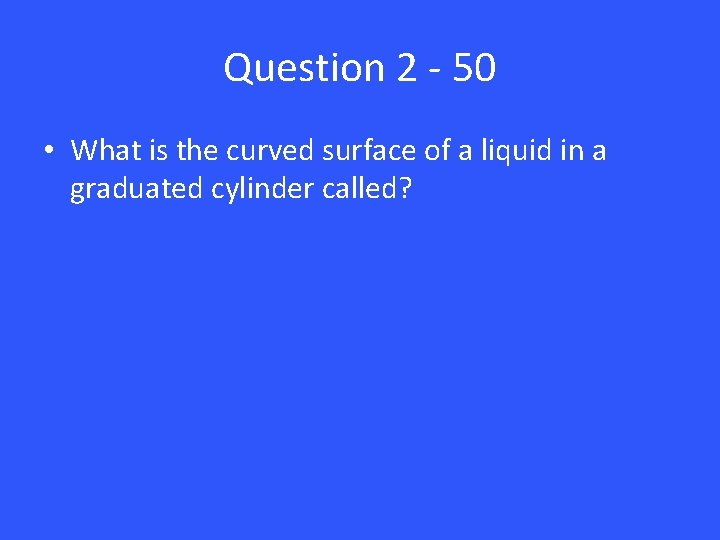Question 2 - 50 • What is the curved surface of a liquid in a graduated cylinder called?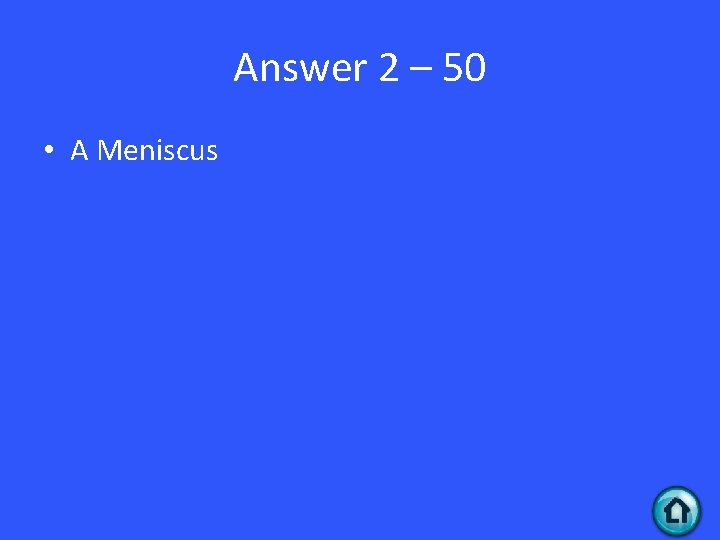Answer 2 – 50 • A MeniscusQuestion 3 - 10 • What formula is used to find the Volume of a Regularly Shaped solid object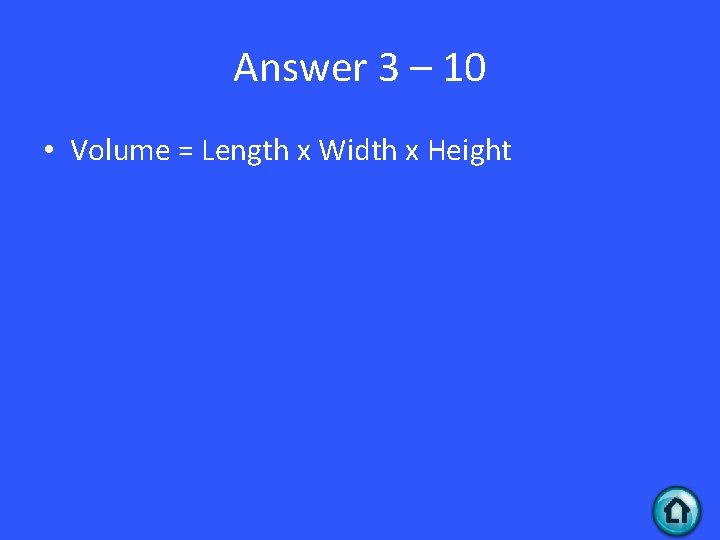Answer 3 – 10 • Volume = Length x Width x Height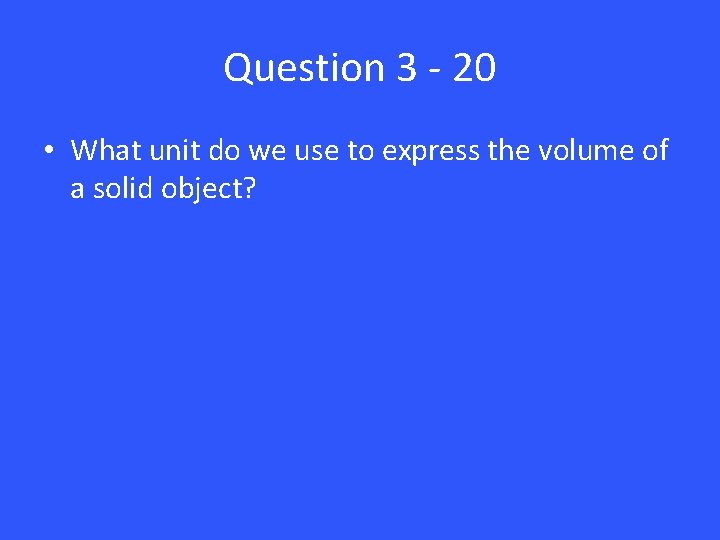Question 3 - 20 • What unit do we use to express the volume of a solid object?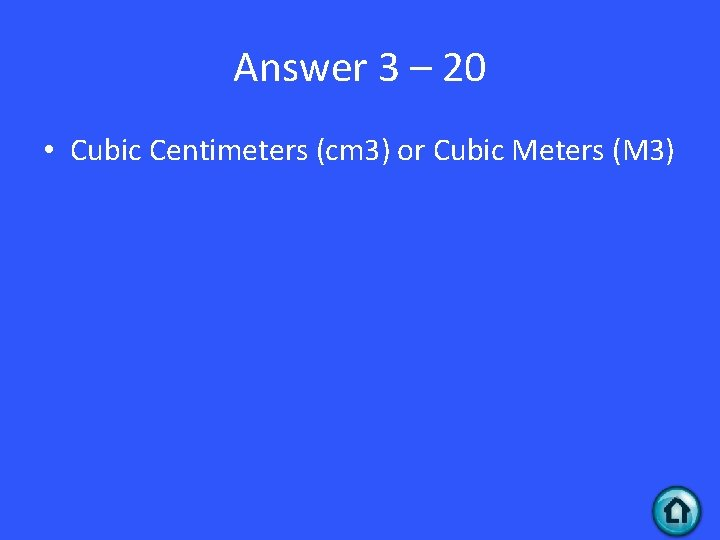Answer 3 – 20 • Cubic Centimeters (cm 3) or Cubic Meters (M 3)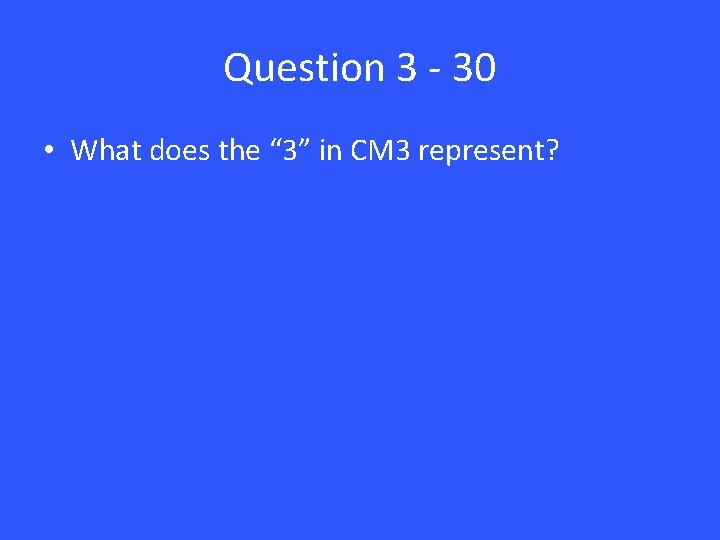Question 3 - 30 • What does the “ 3” in CM 3 represent?Answer 3 – 30 • The three dimensions measured to find the volume. Length, Width and Height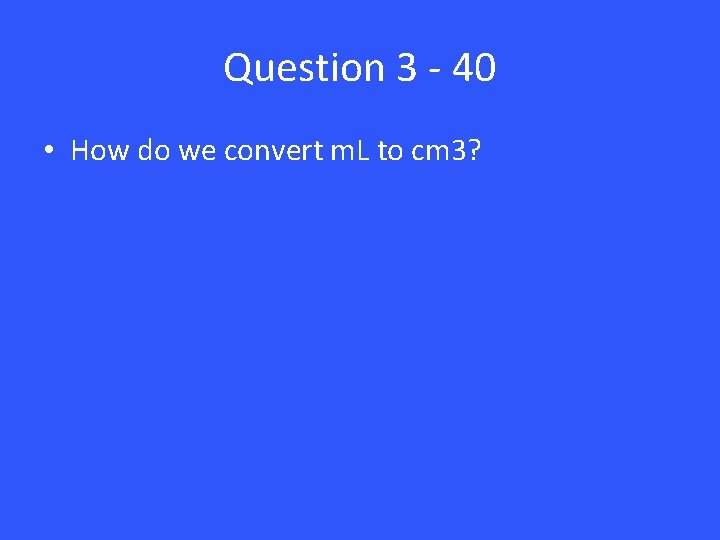Question 3 - 40 • How do we convert m. L to cm 3?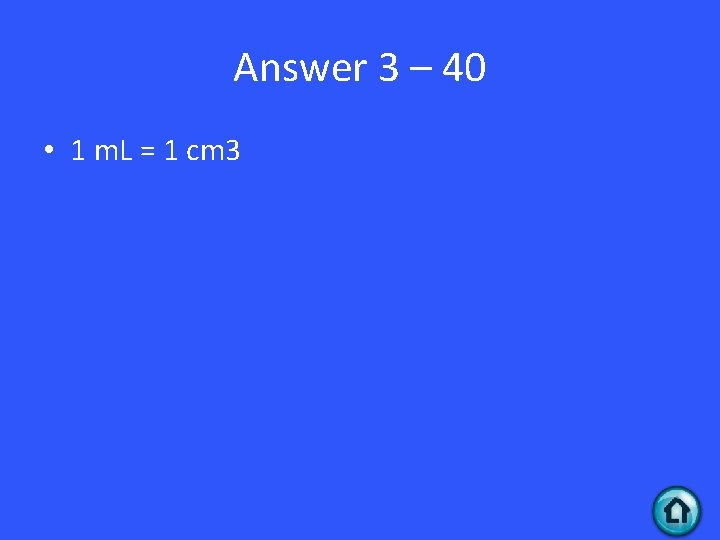Answer 3 – 40 • 1 m. L = 1 cm 3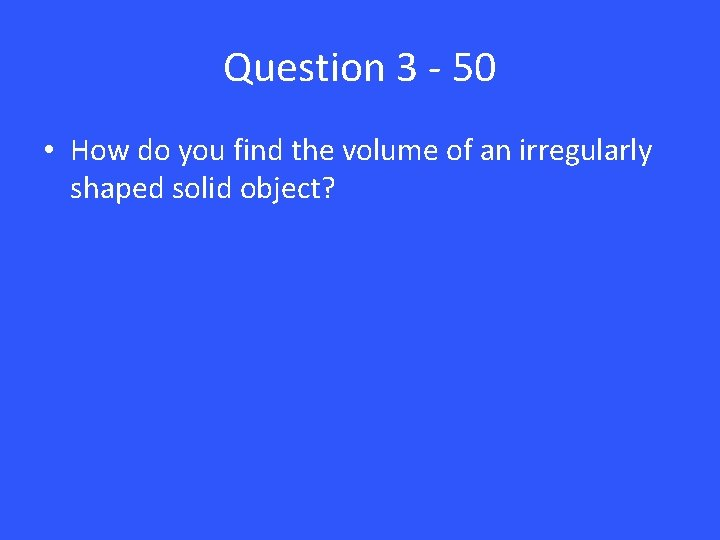Question 3 - 50 • How do you find the volume of an irregularly shaped solid object?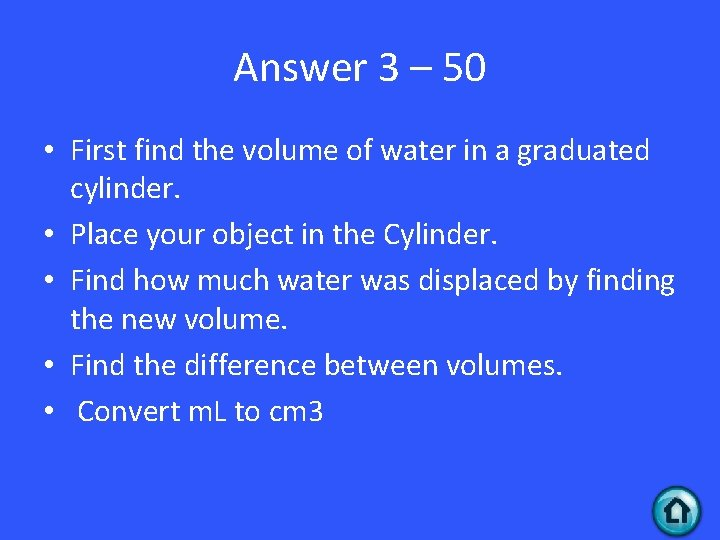Answer 3 – 50 • First find the volume of water in a graduated cylinder. • Place your object in the Cylinder. • Find how much water was displaced by finding the new volume. • Find the difference between volumes. • Convert m. L to cm 3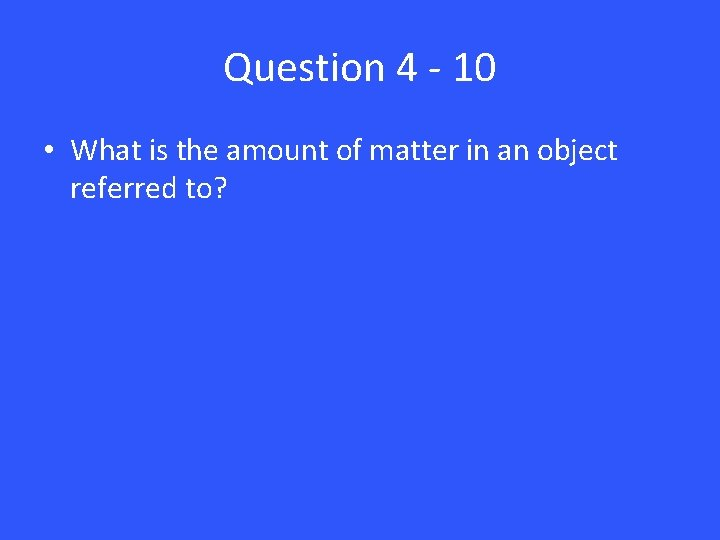Question 4 - 10 • What is the amount of matter in an object referred to?Answer 4 – 10 • MassQuestion 4 - 20 • True or False: If I went to the moon my mass would become less.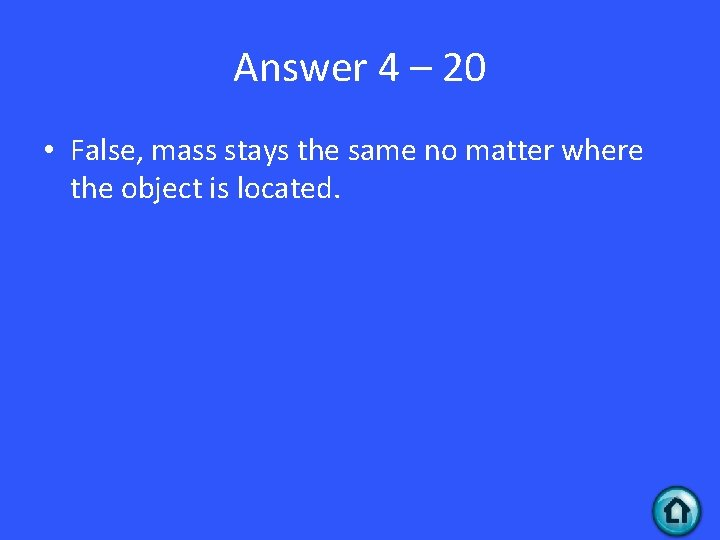Answer 4 – 20 • False, mass stays the same no matter where the object is located.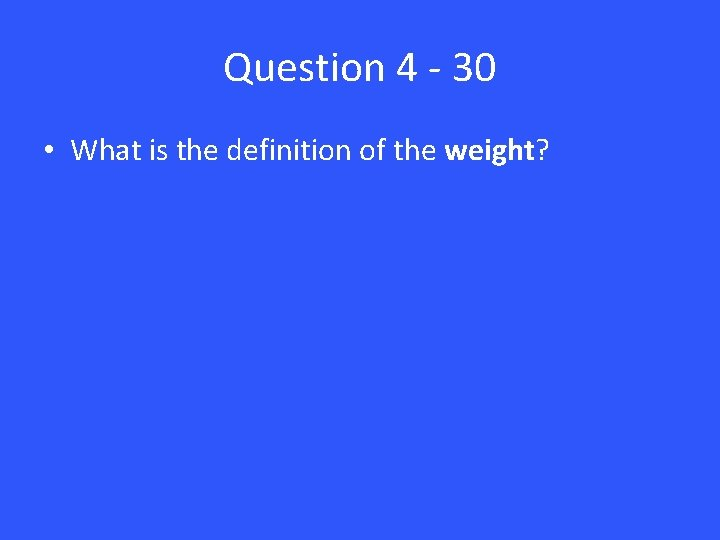Question 4 - 30 • What is the definition of the weight?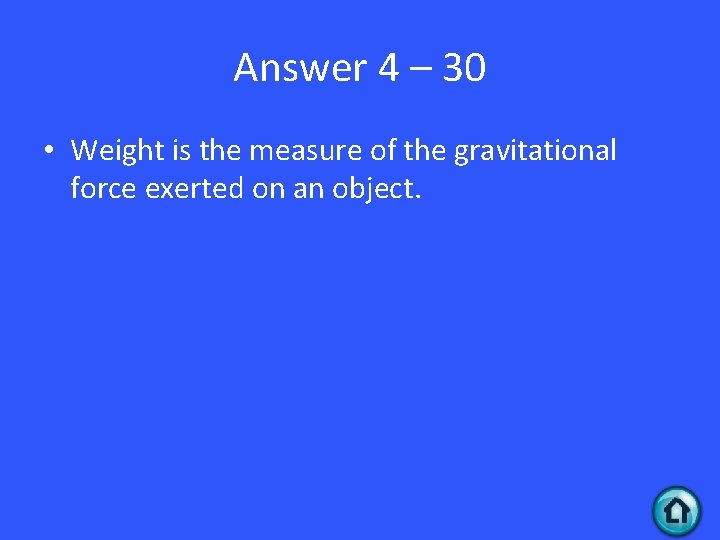Answer 4 – 30 • Weight is the measure of the gravitational force exerted on an object.Question 4 - 40 • What is the relationship between mass and weight?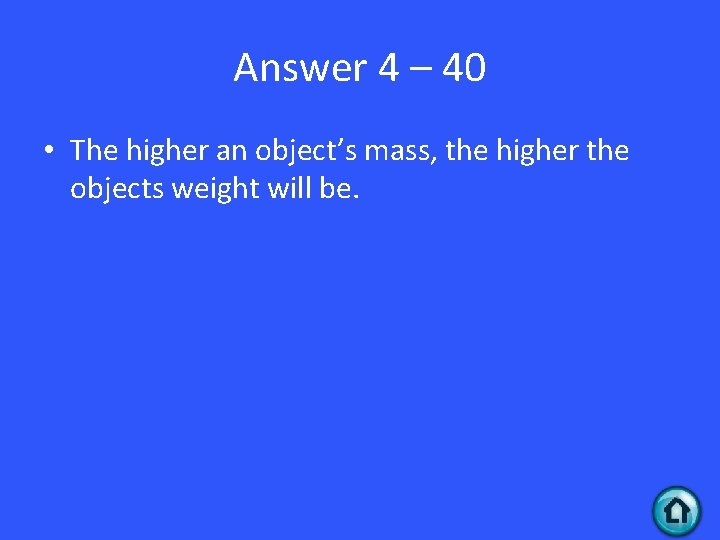Answer 4 – 40 • The higher an object’s mass, the higher the objects weight will be.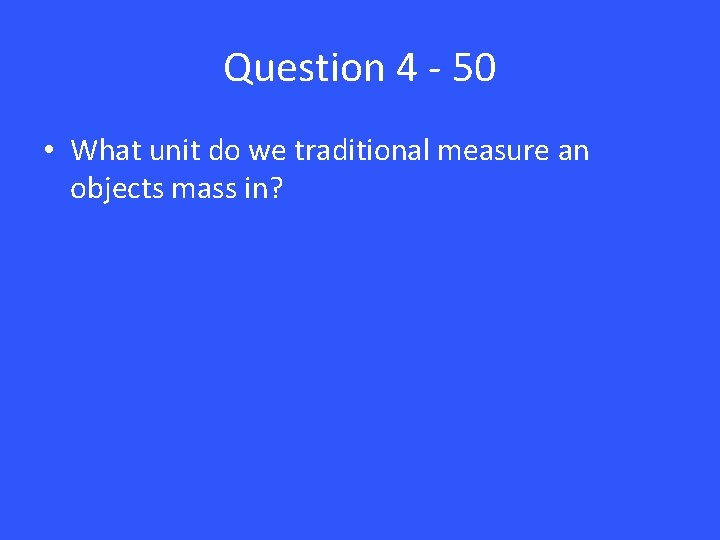Question 4 - 50 • What unit do we traditional measure an objects mass in?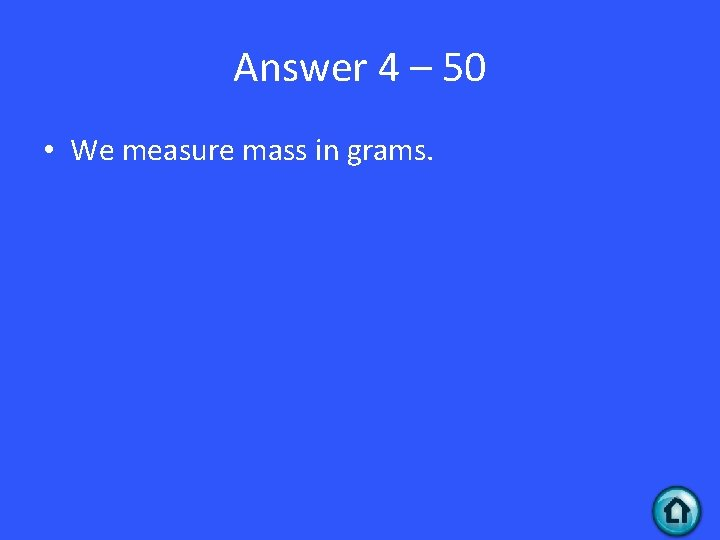Answer 4 – 50 • We measure mass in grams.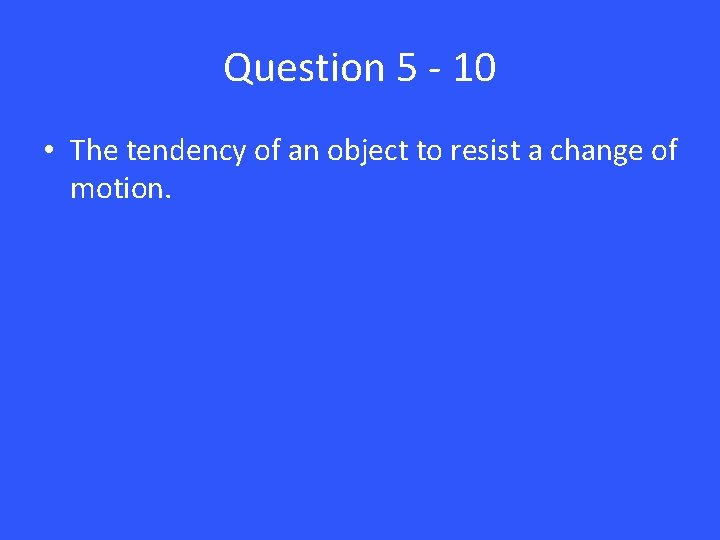Question 5 - 10 • The tendency of an object to resist a change of motion.Answer 5 – 10 • Inertia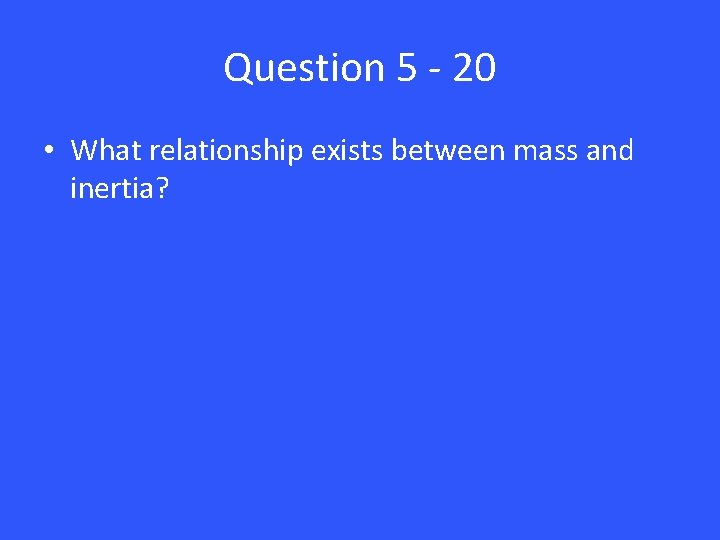Question 5 - 20 • What relationship exists between mass and inertia?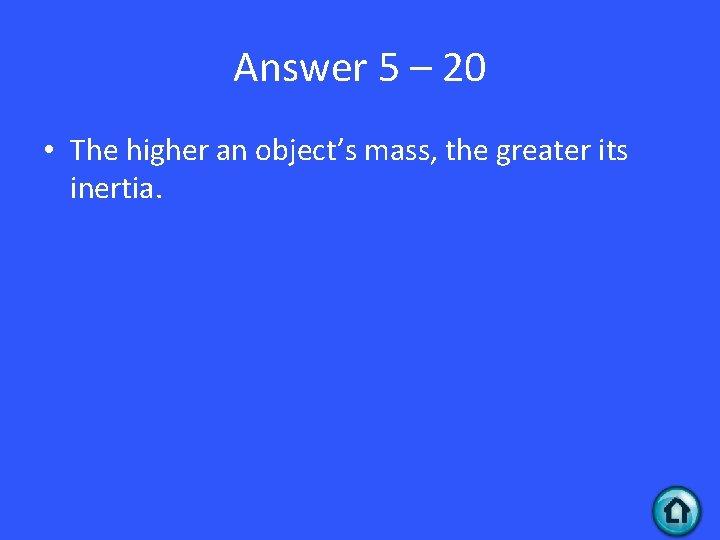Answer 5 – 20 • The higher an object’s mass, the greater its inertia.Question 5 - 30 • What two things must be present for an object to have a weight?Answer 5 – 30 • The object’s mass and a gravitational pullQuestion 5 - 40 • What happens to an object’s weight if we took it to the moon?Answer 5 – 40 • The weight will become less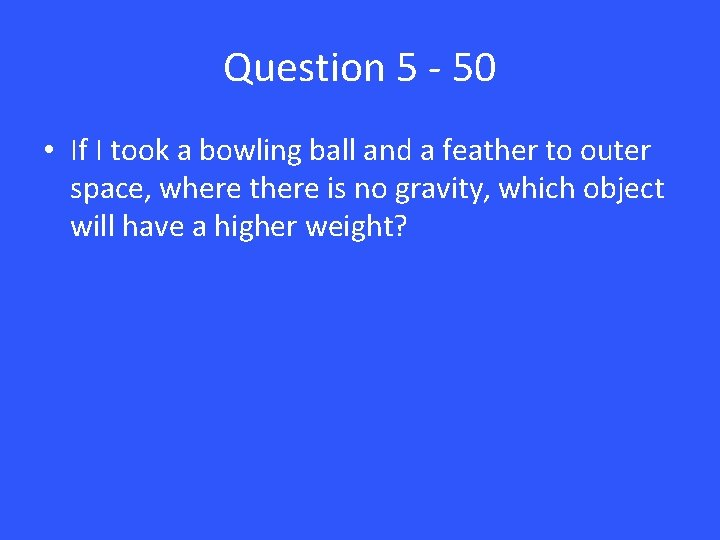Question 5 - 50 • If I took a bowling ball and a feather to outer space, where there is no gravity, which object will have a higher weight?Answer 5 – 50 • Neither, both objects would have a weight of 0 because there is no gravity.